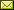## Efficient computation of exact solutions for quantitative model checking

 Sergio Giro (Department of Computer Science, University of Oxford, Oxford, OX1 3QD, UK)

 Quantitative model checkers for Markov Decision Processes typically use finite-precision arithmetic. If all the coefficients in the process are rational numbers, then the model checking results are rational, and so they can be computed exactly. However, exact techniques are generally too expensive or limited in scalability. In this paper we propose a method for obtaining exact results starting from an approximated solution in finite-precision arithmetic. The input of the method is a description of a scheduler, which can be obtained by a model checker using finite precision. Given a scheduler, we show how to obtain a corresponding basis in a linear-programming problem, in such a way that the basis is optimal whenever the scheduler attains the worst-case probability. This correspondence is already known for discounted MDPs, we show how to apply it in the undiscounted case provided that some preprocessing is done. Using the correspondence, the linear-programming problem can be solved in exact arithmetic starting from the basis obtained. As a consequence, the method finds the worst-case probability even if the scheduler provided by the model checker was not optimal. In our experiments, the calculation of exact solutions from a candidate scheduler is significantly faster than the calculation using the simplex method under exact arithmetic starting from a default basis.

In Herbert Wiklicky and Mieke Massink: Proceedings 10th Workshop on Quantitative Aspects of Programming Languages and Systems (QAPL 2012), Tallinn, Estonia, 31 March and 1 April 2012, Electronic Proceedings in Theoretical Computer Science 85, pp. 17–32.
Published: 3rd July 2012.

 ArXived at: https://dx.doi.org/10.4204/EPTCS.85.2 bibtex PDF
References in reconstructed bibtex, XML and HTML format (approximated).
 Comments and questions to:eptcs@eptcs.org For website issues:webmaster@eptcs.org